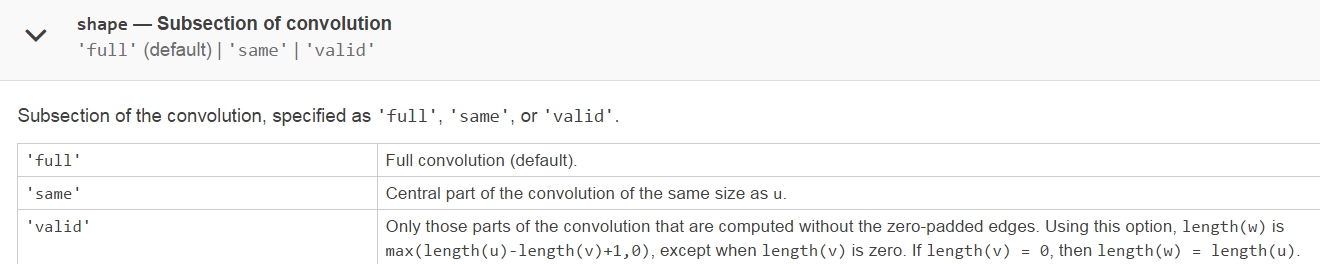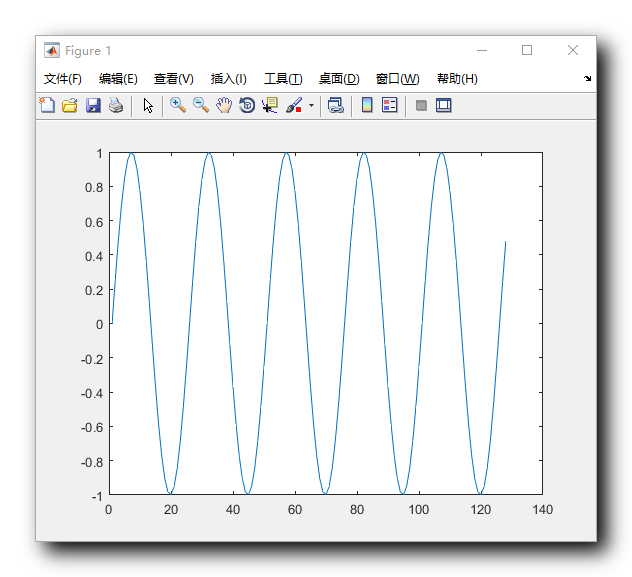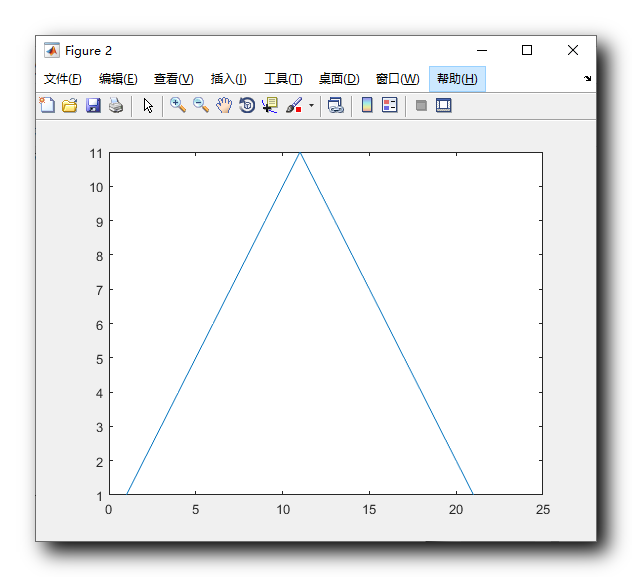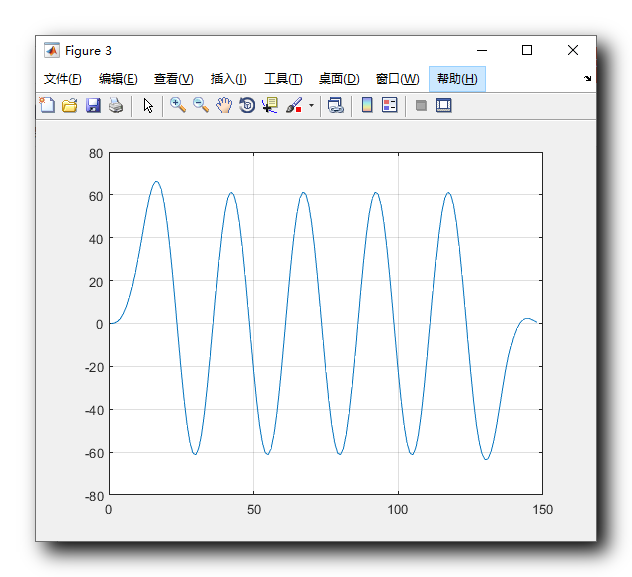• conv是卷积运算，同时也可以做多项式乘法
千次阅读
2016-07-27 08:46:49

conv是卷积运算，同时也可以做多项式的乘法

b=[0.069735,0.388726,0.360530, 0.388726, 0.069735];                 % 原始的系数向量
[sos,G]=tf2sos(b,1);                                                % 原始二阶级联结构的系数向量
bq=brqtize([0.069735,0.388726,0.360530, 0.388726, 0.069735],4)      % 量化后的系数向量
sosq=brqtize(sos,4) ,Gq=brqtize(G,4)                                % 量化后的二阶级联结构的系数向量
subplot(1,2,1),
[H1,w1]=freqz(b,1);plot(w1,abs(H1)),hold on                         % 原始系统的频率特性
[H2,w2]=freqz(bq,1);plot(w2,abs(H2),'-.'),                          % 量化后的系统的频率特性
[H3,w3]=freqz(
conv(sosq(1,1:3),sosq(2,1:3)),1);plot(w3,Gq*abs(H3),':'),% 量化后的二阶级联结构的频率特性

更多相关内容
• 多项式相乘：conv.cpp 【问题描述】 编程实现若干个多项式相乘。多项式的输入输出格式为：系数在前，指数在后，各项按指数递增排列，每个多项式输入时以两个0结束。系数为0的项不输出。例如：1+4X3-9X5 n 输入...

# 多项式相乘：conv.cpp

### 【问题描述】

编程实现若干个多项式相乘。多项式的输入输出格式为：系数在前，指数在后，各项按指数递增排列，每个多项式输入时以两个0结束。系数为0的项不输出。例如：1+4X3-9X5

输入格式可以为：1 0 0 1 0 2 4 3 0 4 -9 5 0 0或者 1 0 4 3 -9 5 0 0

输出只能是：1 0 4 3 -9 5

### 【输入】

输入文件conv.in包含n+1行：第1行为一个正整数n，表示多项式的个数。第2n+1行，每行为一个多项式，多项式输入时以两个0结束。例如：

1 0 1 1 0 0

1 0 -1 1 0 0

1 0 1 2 0 0

表示：(1+x)(1-x)(1+x2)

### 【输出】

输出文件conv.out包含1行，为上述多项式相乘结果。上例的输出为：

1 0 -1 4

表示1-x4

### 【输入输出样例1】

 conv.in conv.out 3 1 0 1 1 0 0 1 0 -1 1 0 0 1 0 1 2 0 0 1 0 -1 4

### 【数据限制】

所有系数、指数均为整数（int类型）。

多项式的个数n满足：2≤n≤20

/*实现n个多项式相乘*/
#include "stdio.h"
const int N0=20;	//常数: 多项式最多20个

typedef struct node{	//多项式的项(链表节点), 数据包含: 项的系数和指数, 下一项的指针.
int c, e;			//c-系数(coefficient); e-指数(exponent)
struct node *next;	//下一个多项式项的指针
}NP;

NP *createPoly(){	//创建一个多项式(对应一个多项式链表)
int c, e;		//c-系数(coefficient); e-指数(exponent)
head=p=new NP;	//初始化多项式的 头指针 & 游标指针
while( true ){
/*(1)输入(获得)多项式'项'的数据[系数+指数]信息 */
scanf("%d%d", &c, &e);	//输入多项式项 ==> 根据输入数据的特点: 系数+指数 紧接着出现!
if( c==0 && e==0 ){	//根据约定, 系数 & 指数 都为0, 则多项式输入结束
break;
}else if(c==0){ //此多项式'项'的系数=0, 可以不需要次多项式'项'!
continue;
}

/*(2)多项式数据项有效, 则创建 多项式'项'(对应于链表节点)*/
p->next=new NP;
p=p->next;

/*(3)维护多项式项的数据信息*/
p->c=c;
p->e=e;
}
p->next=NULL; //多项式最后一个节点的 "下一个多项式项 指针"为空 ==> 标志为多项式(链表)末尾

}

void printPoly( NP *head ){ //打印输出多项式链表
while( p ){
printf("%d %d ", p->c, p->e);
p=p->next;
}
printf("\n");
}

NP *p; //p-遍历指针
delete p;
}
}

NP *addPoly( NP *ha, NP *hb){ //实现两个多项式相加(需要处理同类项[即指数相同的项]),返回结果多项式的链头指针.
NP *hc, *pc, *pa=ha->next, *pb=hb->next;
hc=pc=new NP;
int c, e;
while( pa || pb )
{
if( pa && (pb==NULL  || pa->e

e)  )
{	c=pa->c;
e=pa->e;
pa=pa->next;
}
else if( pb && (pa==NULL  || pb->e

e)  )
{	c=pb->c;
e=pb->e;
pb=pb->next;
}
else
{	c=pa->c+pb->c;
e=pa->e;
pa=pa->next;
pb=pb->next;
}
if( c )
{	pc->next=new NP;
pc=pc->next;
pc->c=c;
pc->e=e;
}
}
pc->next=NULL;
return hc;

}

NP *oneXmulty( NP *pa, NP *hb ){ //pa-某个多项式'项'; hb-一个多项式(多个'项'), 返回pa*ha的结果多项式:头指针
NP *hc, *pc, *pb=hb->next;
hc=pc=new NP;
while( pb )
{
pc->next=new NP;
pc=pc->next;
pc->c=pa->c*pb->c;
pc->e=pa->e+pb->e;
pb=pb->next;
}
pc->next=NULL;
return hc;
}
NP *multyXmulty( NP *ha, NP *hb )
{	NP *hc, *ht, *pa=ha->next;
hc=new NP;
hc->next=NULL;
while( pa )
{
ht=oneXmulty( pa, hb );
freePoly( ht );
pa=pa->next;
}
return hc;

}

int main(){
int n, i; //n-多项式的个数; i-循环控制变量
NP *hink[N0], *hc, *temp;	//hink[]-待相乘的若干个多项式的头指针; hc-多项式相乘结果头指针;
scanf("%d", &n);	//输入多项式的个数n

/*(1)创建n个多项式链表*/
for(i=0;i展开全文算法
• convConvolution and polynomial multiplicationSyntaxw = conv(u,v)w = conv(u,v,shape)...如果u和v是多项式系数的向量，则对它们进行卷积相当于将两个多项式相乘。w = conv(u,v,shape)returns a subsection of the...

conv

Convolution and polynomial multiplication

Syntax

w = conv(u,v)

w = conv(u,v,shape)

Description

w = conv(u，v)返回向量u和v的卷积。如果u和v是多项式系数的向量，则对它们进行卷积相当于将两个多项式相乘。

w = conv(u,v,shape) returns a subsection of the convolution, as specified by shape. For example, conv(u,v,'same') returns only the central part of the convolution, the same size as u, and conv(u,v,'valid') returns only the part of the convolution computed without the zero-padded edges.

w = conv(u，v，shape)返回卷积的子部分，由形状指定。

例如，conv(u，v，'same')仅返回卷积的中心部分，与u的大小相同，而conv(u，v，'valid')仅返回计算后的卷积部分而没有零填充边。

Polynomial Multiplication via Convolution

Create vectors u and v containing the coefficients of the polynomials x^2 + 1 and 2x + 7.

u = [1 0 1];

v = [2 7];

Use convolution to multiply the polynomials.

w = conv(u,v)

w = 1×4

2 7 2 7

w contains the polynomial coefficients for  2x^3 + 7x^2 + 2x + 7.

Vector Convolution

Create two vectors and convolve them.

u = [1 1 1];

v = [1 1 0 0 0 1 1];

w = conv(u,v)

w = 1×9

1 2 2 1 0 1 2 2 1

The length of w is length(u)+length(v)-1, which in this example is 9.

Central Part of Convolution

Create two vectors. Find the central part of the convolution of u and v that is the same size as u.

u = [-1 2 3 -2 0 1 2];

v = [2 4 -1 1];

w = conv(u,v,'same')

w = 1×7

15 5 -9 7 6 7 -1

w has a length of 7. The full convolution would be of length length(u)+length(v)-1, which in this example would be 10.展开全文• 一、Matlab 卷积和多项式乘法 conv 函数、 二、使用 matlab 代码求卷积并绘图

# 一、Matlab 卷积和多项式乘法 conv 函数

Matlab 文档地址 : https://ww2.mathworks.cn/help/matlab/ref/conv.html

函数语法 :

// 返回向量 u 和 v 的卷积。如果 u 和 v 是多项式系数的向量，对其卷积与将这两个多项式相乘等效。
w = conv(u,v)
// 返回如 shape 指定的卷积的分段。
// 例如，conv(u,v,'same') 仅返回与 u 等大小的卷积的中心部分，
// 而 conv(u,v,'valid') 仅返回计算的没有补零边缘的卷积部分。
w = conv(u,v,shape)


u u 是 " 输入序列 " , v v 是 " 单位脉冲响应 " ;

# 二、使用 matlab 代码求卷积并绘图

求下面的 " 线性时不变系统 " 的 输出序列 ;

输入序列 : x ( n ) = s i n ( 2 π f 0 n / F s ) ,   0 ≤ n ≤ 127 x(n) = sin(2\pi f_0n/F_s) , \ 0 \leq n \leq 127 ,

f 0 = 0.4 k H z , F s = 10 k H z f_0 = 0.4kHz , F_s = 10kHz

单位脉冲响应 :

h ( n ) = { 1 , 2 , 3 , 4 , 5 , 6 , 7 , 8 , 9 , 10 , 11 , 10 , 9 , 8 , 7 , 6 , 5 , 4 , 3 , 2 , 1 } h(n) = \{ 1,2,3,4,5,6,7,8,9,10,11,10,9,8,7,6,5,4,3,2,1 \}

上述系统的输出就是 " 输入序列 " 与 " 输出序列 " 的卷积 ;

f 0 = 0.4 k H z f_0 = 0.4kHz 表示模拟信号频率是 0.4 k H z 0.4kHz ,

F s = 10 k H z F_s = 10kHz 表示数字采样频率是每秒采集 10 k 10k 个样本 , 也就是 10000 10000 个样本 ;

x ( n ) x(n) 默认是一个无限序列 , 为了方便计算 , 这里为其加了一个区间 0 ≤ n ≤ 127 0 \leq n \leq 127 ;

数字频率计算 :

数字频率 ( 单位 Hz ) : f = f 0 / F s = 0.4 / 10 = 0.04 f = f_0 / F_s = 0.4 / 10 = 0.04

matlab 代码示例 :

%清除内存
clear;

%输入序列 , 模拟信号频率 0.4kHz , 采样频率 10kHz
% n 的取值范围是 0 到 127 闭区间整数序列
x=sin(2 * pi * 0.4 * (0:127) / 10);

%单位脉冲响应
%1,2,3,4,5,6,7,8,9,10,11,10,9,8,7,6,5,4,3,2,1
%1 ~ 10 索引的序列就是 1,2,3,4,5,6,7,8,9,10
h(1:10)=(1:10);
%11 索引的序列是 11
h(11)=11;
%12 ~ 21 索引的序列是 10,9,8,7,6,5,4,3,2,1
h(12:21)=(10:-1:1);

%求 " 单位脉冲响应 " 序列的频谱
hf = freqz(h, 1, 1024);
%求 " 输入序列 " 的频谱
xf = freqz(x, 1, 1024);

%求卷积 , 线性时不变系统的输出序列就是 输入序列 与 单位脉冲响应 之间的卷积
y=conv(h,x);

%建立幕布
figure;
%绘制 "输入序列" 图像
plot(x);

%建立幕布
figure;
%绘制 "单位脉冲响应" 图像
plot(h);

%建立幕布
figure;
%绘制 y 函数的值 , 也就是卷积计算结果
plot(y);

% 打开网格
grid on;


输入序列 x ( n ) x(n) 的函数图像如下 :单位脉冲响应 h ( n ) h(n) 函数图像如下 :输出序列 y ( n ) y(n) 图像如下 :展开全文matlab 数字信号处理 卷积
• 1.从多项式乘法到卷积和 这里有两个多项式： 两个多项式相乘 看一下h(x)的0次方项的系数是怎么算的 你会发现，在求c0时，这个等式的右边的每一项都是数列中的某一项和数列中的某一项相乘，而且任何一对...卷积
• % 多项式运算训练 conv poly deconv roots polyval polyft% 初始化clc;...% conv - 卷积同时适应多项式乘法x = [ 2,3 ];y = [ 1 0 9 ];z = conv( x,y );% z =%% 2 3 18 27% poly - 由根求多项式y1 = [ 2,3 ];y2 = ...
• ## 多项式乘法运算终极版

万次阅读 多人点赞 2014-09-05 17:15:36
换来求多项式乘法。可以发现它是利用了单位复根的特殊性质，大大减少了运算，但是这种做法是对复数系数的矩阵 加以处理，每个复数系数的实部和虚部是一个正弦及余弦函数，因此大部分系数都是浮点数，我们必须做...
• 文章目录1 多项式的建立2 多项式的求值与求根3 多项式乘法和除法4 多项式的导数和积分5 多项式展开6 多项式拟合 1 多项式的建立 n次多项式用一个n+1维的行向量表示（幂次由高到低） % 1.1 直接输入系数法建立多项式...matlab
• 多项式的表达与创建MATLAB中用一维行向量来表示多项式，将多项式的系数按照降幂次序存放在向量中。请注意上面一句话，这将是MATLAB中对多项式操作的关键MATLAB中对多项式中缺少的幂次的系数应补充为0，不能空过去例...
• 1、多项式的表达式和创建 MATLAB中使用一维向量来表示多项式。将多项式按照降幂次序存放在向量中。 多项式就可以用向量 [2 3 5 0 1]来表示。 2、多项式求根 &gt;&gt; p=[1 2 1] p = 1 2 1 &gt...
• 多项式 多项式的表示 一个p阶的多项式可以用一个含有p+1个元素的向量表示，MATLAB表示多项式为...多项式乘法。 p1=[1 1];%它是x+1的系数矩阵 p2=[1 0];%它是x的系数矩阵 P=conv(p1,p2) P= 1 1 0 %P=[1 1 0]，说...matlab
• matlab的应用-多项式... 多项式函数主要有： roots 求多项式的根 poly 特征多项式 polyval 多 项式的计算 poly2str(p, x )多项式代换 polyfit 多项式曲线拟合 conv 多项式乘法 deconv 多项式除法 polyder 微分多项...
• ## MATLAB之多项式计算

万次阅读 多人点赞 2018-05-30 21:36:11
2、多项式乘法乘法conv函数，例如：conv(p1,p2), p1、p2为两个多项式的系数向量，计算结果为p1*p2的新的向量的值。3、多项式除法： [Q,r]=deconv(p1,p2),多项式除法，Q返回p1/p2的商式，...
• conv(向量卷积运算) 两个向量卷积，简单理解其实就是多项式乘法。 比如:p=[1 2 3]，q=[1 1]是两个向量，p和q的卷积计算方法如下： 把p的元素作为一个多项式的系数，多项式按升幂(或降幂)排列，比如就按升幂吧，写出...
• 1.用C语言实现matlab中conv（）自带的函数 int max(int a,int b) { return a>b ? a:b; } int min(int a, int b) { return a<b ? a:b; } void conv(double *Ptr_Src1,double *Ptr_Src2, int Src1Lenth, int...matlab
• 多项式的表示： ...只知道多项式的零点，可以用poly函数来创建多项式的系数数组 poly函数的输入还可以是二维数组，此时返回的值为数组的特征多项式|lamdaE-A| 多项式求值： p=[2 -3 0 -1]； a=1.6 %...matlab 数学
• conv(A,B):计算多项式的乘法，其中A,B为多项式系数组成的向量，返回值为多项式乘法结果组成的向量。 >> conv([2,1,3],[1,2,3]) ans = 2 5 11 9 9 [Q,r]=deconv(A,B)：计算多项式的除法，其中A,B为...matlab 矩阵
•matlab 矩阵 算法
• MATLAB能够很好的解决多项式运算问题，这些函数用于多项式的建立、多项式求值、多项式乘法和除法、多项式求导、多项式展开和拟合等等。插值函数，主要包括一维插值、二维插值、样条插值和高维插值等内容。MATLAB提供...
• conv(向量卷积运算)两个向量卷积，简单理解其实就是多项式乘法。比如:p=[1 2 3],q=[1 1]是两个向量，p和q的卷积计算方法如下：把p的元素作为一个多项式的系数，多项式按升幂(或降幂)排列，比如就按升幂吧，写出对应...
• ## matlab中conv函数的使用和理解

万次阅读 多人点赞 2020-12-29 09:54:55
conv 计算 卷积和多项式乘法 w = conv(u,v) 返回向量 u 和 v 的卷积。如果 u 和 v 是多项式系数的向量，对其卷积与将这两个多项式相乘等效。 w = conv(u,v,shape) 返回如 shape 指定的卷积的分段。例如，conv(u,v,...matlab
• 一、对多项式f(x)与g(x)做加、减、乘、除运算，并计算它们的商的导函数。 步骤： ①输入变量 ②进行计算 ③得出答案 >> f=[3,0,-6,0,1]; >> g=[0,0,1,0,-1]; >> f+g; %%加法 >> f-g; %%减法 >> conv(f,g); %%乘法 >...
• 函数用于求特征多项式。该命令会创造一个多项式，其分量为多项式系数，该多项式具有给定的多项式的根“A”。　poly(A)当A是一个N*N矩阵式，poly(A)命令求出A的特征多项式　det(lambda*eye(size(A))-A) 当V是向量时...
• 1.多项式求根在MATLAB中求取多项式的根用roots函数函数:roots功能:一元高次方程求解。语法:roots(c)说明:返回一个列向量，其元素为多项式c的解。例:求方程x3-8x2+6x–30=0的解。c = [0 -8 6 30]; %注意需包含所有...
• 1、多项式的表示 在MATLAB中创建多项式向量时，注意三点: ...(2)多项式乘法 conv (P1,P2):多项式相乘函数。 在这里，P1、P2是两个多项式系数向量。 (3）多项式除法 [Q,r]=deconv(P1,P2):多项式相除函数。 其中，Q返.
• 多项式计算 roots ployval MATLAB
• matlab中conv函数简介 1、conv函数：用于计算向量的卷积和多项式乘法。 2、用法说明 实例1：多项式乘法，（s^2+2s+2）（s+4）（s+1）可以使用w=conv（[1,2,2]，conv（[1,4]，[1,1]）） 实例2：向量的卷积，长度为m...matlab c++ 卷积...